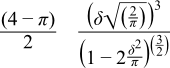#Boost C++ Libraries

...one of the most highly regarded and expertly designed C++ library projects in the world.

This is the documentation for an old version of boost. Click here for the latest Boost documentation.
##### Skew Normal Distribution

`#include <boost/math/distributions/skew_normal.hpp>`

```namespace boost{ namespace math{

template <class RealType = double,
class Policy   = policies::policy<> >
class skew_normal_distribution;

typedef skew_normal_distribution<> normal;

template <class RealType, class Policy>
class skew_normal_distribution
{
public:
typedef RealType value_type;
typedef Policy   policy_type;
// Constructor:
skew_normal_distribution(RealType location = 0, RealType scale = 1, RealType shape = 0);
// Accessors:
RealType location()const; // mean if normal.
RealType scale()const; // width, standard deviation if normal.
RealType shape()const; // The distribution is right skewed if shape > 0 and is left skewed if shape < 0.
// The distribution is normal if shape is zero.
};

}} // namespaces
```

The skew normal distribution is a variant of the most well known Gaussian statistical distribution.

The skew normal distribution with shape zero resembles the Normal Distribution, hence the latter can be regarded as a special case of the more generic skew normal distribution.

If the standard (mean = 0, scale = 1) normal distribution probability density function isand the cumulative distribution functionthen the PDF of the skew normal distribution with shape parameter α, defined by O'Hagan and Leonhard (1976) isGiven location ξ, scale ω, and shape α, it can be transformed, to the form:and CDF:where T(h,a) is Owen's T function, and Φ(x) is the normal distribution.

The variation the PDF and CDF with its parameters is illustrated in the following graphs:##### Member Functions
```skew_normal_distribution(RealType location = 0, RealType scale = 1, RealType shape = 0);
```

Constructs a skew_normal distribution with location ξ, scale ω and shape α.

Requires scale > 0, otherwise domain_error is called.

```RealType location()const;
```

returns the location ξ of this distribution,

```RealType scale()const;
```

returns the scale ω of this distribution,

```RealType shape()const;
```

returns the shape α of this distribution.

(Location and scale function match other similar distributions, allowing the functions `find_location` and `find_scale` to be used generically).

NoteWhile the shape parameter may be chosen arbitrarily (finite), the resulting skewness of the distribution is in fact limited to about (-1, 1); strictly, the interval is (-0.9952717, 0.9952717). A parameter δ is related to the shape α by δ = α / (1 + α²), and used in the expression for skewness##### Non-member Accessors

All the usual non-member accessor functions that are generic to all distributions are supported: Cumulative Distribution Function, Probability Density Function, Quantile, Hazard Function, Cumulative Hazard Function, mean, median, mode, variance, standard deviation, skewness, kurtosis, kurtosis_excess, range and support.

The domain of the random variable is -[max_value, +[min_value]]. Infinite values are not supported.

There are no closed-form expression known for the mode and median, but these are computed for the

• mode - by finding the maximum of the PDF.
• median - by computing `quantile(1/2)`.

The maximum of the PDF is sought through searching the root of f'(x)=0.

Both involve iterative methods that will have lower accuracy than other estimates.

##### Testing

The R Project for Statistical Computing using library(sn) described at Skew-Normal Probability Distribution, and at R skew-normal(sn) package.

Package sn provides functions related to the skew-normal (SN) and the skew-t (ST) probability distributions, both for the univariate and for the the multivariate case, including regression models.

Wolfram Mathematica was also used to generate some more accurate spot test data.

##### Accuracy

The skew_normal distribution with shape = zero is implemented as a special case, equivalent to the normal distribution in terms of the error function, and therefore should have excellent accuracy.

The PDF and mean, variance, skewness and kurtosis are also accurately evaluated using analytical expressions. The CDF requires Owen's T function that is evaluated using a Boost C++ Owens T implementation of the algorithms of M. Patefield and D. Tandy, Journal of Statistical Software, 5(5), 1-25 (2000); the complicated accuracy of this function is discussed in detail at Owens T.

The median and mode are calculated by iterative root finding, and both will be less accurate.

##### Implementation

In the following table, ξ is the location of the distribution, and ω is its scale, and α is its shape.

Function

Implementation Notes

pdf

Using:cdf

Using:where T(h,a) is Owen's T function, and Φ(x) is the normal distribution.

cdf complement

Using: complement of normal distribution + 2 * Owens_t

quantile

Maximum of the pdf is sought through searching the root of f'(x)=0

quantile from the complement

-quantile(SN(-location ξ, scale ω, -shapeα), p)

location

location ξ

scale

scale ω

shape

shape α

median

quantile(1/2)

meanmode

Maximum of the pdf is sought through searching the root of f'(x)=0

varianceskewnesskurtosis

kurtosis excess-3

kurtosis excessCopyright © 2006-2010 John Maddock, Paul A. Bristow, Hubert Holin, Xiaogang Zhang, Bruno Lalande, Johan Råde, Gautam Sewani, Thijs van den Berg and Benjamin Sobotta Distributed under the Boost Software License, Version 1.0. (See accompanying file LICENSE_1_0.txt or copy at http://www.boost.org/LICENSE_1_0.txt)